• 关系运算符与赋值运算符
千次阅读
2021-06-11 22:54:38
package 运算符;

public class Demo3 {//运算符
/**
* 算术运算符：+，-，*，/，%（模运算）,++,--
* 赋值运算符:=(a = 10; 把10赋值给a)
* 关系运算符:>,<,>=,<=,==,!=(不等于)
* 逻辑运算符:&&(与),||(或),!(非) 与或非
* 位运算符:&,|,^,~,>>,<<,>>>
*/
public static void main(String[] args) {
//二元运算符(Ctrl+D复制当前行到下一行)
int a = 10;
int b = 20;
int c = 25;
int d = 25;
System.out.println(a+b);
System.out.println(a-b);
System.out.println(a*b);
System.out.println(a/b);//0.5四舍五入要强制转换
System.out.println(a/(double)b);

System.out.println("-------------------------------------");
long e = 12123123412345L;
int f = 123;
short g = 10;
byte h = 8;
System.out.println(e+f+g+h);//long 有一个数为long结果为long
System.out.println(f+g+h);//int 如果没Long结果都为int无论有没有int
System.out.println(g+h);//int

long z =10000;
int y = 1000000;
System.out.println(z+y);//Long
System.out.println("-------------------------------------");

/**关系运算符
* >大于
* >=大于等于
* <小于
* <=小于等于
* ==等于
* !=不等于
* =赋值运算符
* ==关系运算符
* 关系运算符的运算结果一定是布尔类型：true/false
*
*/
int i = 10;
int j = 20;
int r = 22;
System.out.println(r%i);//c除a 22除10=2余2
System.out.println(i>j);//false
System.out.println(j>i);//true
System.out.println(i==j);//false
// 注意 "="赋值运算符 "=="等于
//变量a中的值是否等于变量b中的值
//变量中保存的值之间的大小比较
System.out.println(i!=j);//true
//false错 true对

/**
* 逻辑运算符：
* & 逻辑与【并且】（两边的算子都是true，结果才是true）
* | 逻辑或【或者】（两边的算子只要有一个是true，结果就是true）
* ! 逻辑非（取反，！false就是true，！true就是false，这就是一个单目运算符）
* ^ 逻辑异或 （两边的算子只要不一样，结果就是true）
* && 短路与
* || 短路或
*特点：
*  1.逻辑运算符要求两边的算子都是布尔类型，
*  并且逻辑运算符最终的运算结果也是布尔类型。
*  2.短路与和逻辑与最终的运算结果是相同的，
*  只不过短路与存在短路现象。
*  3.短路或和逻辑或最终的运算结果是相同的，
*  只不过短路或存在短路现象。
*/
//运算符优先级不确定加小括号
System.out.println("----");
System.out.println(5 > 3 & 5 > 2);//true
System.out.println(5 > 3 |  5 > 6);//true

System.out.println(true & true );//true
System.out.println(true & false );//false
System.out.println(false & false );//false

System.out.println(true | false);//true
System.out.println(false | false);//false

System.out.println(true ^ false);//true
System.out.println(false ^ false);//false
System.out.println(true ^ true);//false

}

}

package 运算符;

public class Demo4 {
public static void main(String[] args) {
//赋值运算符
/**
* ++自增 --自减 一元运算符
*/
//下面以++为例
//关于++运算符【自加1】
int k =10;
//++运算符可以出现在变量后面
k++;

System.out.println(k);//11
int y = 10;
//++运算符可以出现在变量前面
++y;
System.out.println(y);//11
/**
* //++运算符可以出现在变量钱，也可以出现在变量后，无论是变量前还是变量后，
* 只要++运算结束，该变量中的值一定会自加1
*/

//++出现在变量后
//规则：先做赋值运算，在对变量中保存的值在自增1
int a = 100;
int b = a++;
System.out.println(a);//a=101
System.out.println(b);//100

System.out.println("-------------");

//举例
int e = 100;
int r = e++;//e=101 r=100
//先赋值 r = e =100 ；再自增 e++ e+1=101
System.out.println(e);
System.out.println(r);

System.out.println("-------------");

//++出现在在变量前
//规则：先进行自加1运算，然后在进行赋值
int m = 20;
int n = ++m;
System.out.println(m);//21
System.out.println(n);//21

System.out.println("-------------");

//举例
int o = 57;
int p = ++o;
System.out.println(o);
System.out.println(p);//o=58 p=58
//p=++o o先自加1 ++o 57+1=58 在赋值 p=o=58

}
}java
更多相关内容
• 文章目录算术运算符关系运算符逻辑运算符赋值运算符 算术运算符 算术运算符有：+ - * / % ++ – ++分为前置++ 和后置++，这俩者有所不同。 前置++是先加后用，后置++是先用后加。 前置–是先加后用，后置–是先用后...

# 算术运算符

算术运算符有：+ - * / % ++ –
++分为前置++ 和后置++，这俩者有所不同。
前置++是先加后用，后置++是先用后加。
前置–是先加后用，后置–是先用后加。
如下：我们可以看出++a在a++之前，也就是说 ++a和a++屏幕显示的是6。

public class s{
public static void main(String[] args)
{

int a,b;
a=5;b=15;
System.out.println(a+b);
System.out.println(a-b);
System.out.println(a*b);
System.out.println(a/b);
System.out.println(a%b);
System.out.println(++a);
System.out.println(a++);

}
}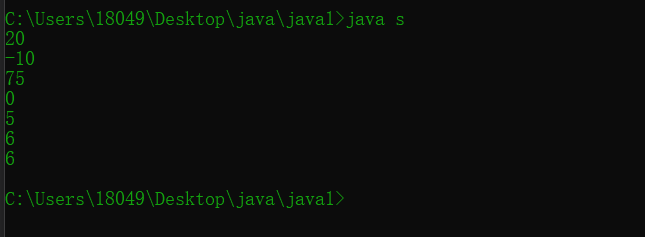## 关系运算符

关系运算符:> >= < <= == !=
关系运算符返回的值类型是boolean类型，即true和false

public class s{
public static void main(String[] args)
{

int a,b;
a=15;b=15;
System.out.println(a>b);
System.out.println(a>=b);
System.out.println(a<b);
System.out.println(a<=b);
System.out.println(a==b);
System.out.println(a!=b);

}
}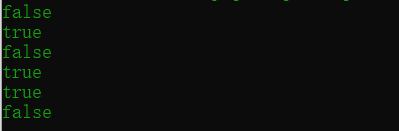### 逻辑运算符

逻辑运算符有： &（与） |（或） ^（异或） !（非）
&&(短路与) ||（短路或）
&&和&都是一假即假
||和|都是一真即真
^只有俩边不同才为真
！真变成假，假变成真
&&和&有所区别，当&前面的表达式为假的时候，后面的表达式依然会进行运算，而&&不会。

public class s{
public static void main(String[] args)
{

int a,b;
a=15;
b=15;
System.out.println(true&false);
System.out.println(true&true);
System.out.println(true|false);
System.out.println(true^false);
System.out.println(true^true);
System.out.println(!true);
System.out.println(a>b&&a++>b);
System.out.println(a);
System.out.println(a>b&a++>b);
System.out.println(a);

}
}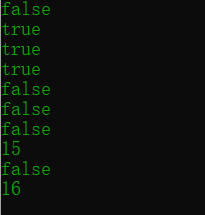可以看出&&后面的表达式并没有执行，所以a依然为15，
而&后面的表达式执行，a为16.

### 赋值运算符

赋值运算符：= += -= *= /= %=

public class s{
public static void main(String[] args)
{

byte a=10;
a=a+4;

}
}


这样的代码编译会报错，因为可能会损失精度，所以需要加强制类型转换。
而这样不会：

public class s{
public static void main(String[] args)
{

byte a=10;
a+=4;

}
}


这说明复合运算符会默认加上强制类型转换，即a=(byte)(a+4)。

展开全文javase
• 算术运算符、关系运算符、逻辑运算符、赋值运算符详解优先级关系一、算术运算符二、关系运算符三、逻辑运算符四、赋值运算符 四种运算符优先级关系： 算术运算符>关系运算符>逻辑运算符>赋值运算符 一...

### 算术运算符、关系运算符、逻辑运算符、赋值运算符代码详解与优先级关系

四种运算符优先级关系：

算术运算符>关系运算符>逻辑运算符>赋值运算符

# 一、算术运算符代码举例：

public static void main(String[] args){
//除号： /
int q = 12;
int w = 5;
double result1 = q / w;// 2.0
double result3 = q / (w + 0.0);// 2.4
double result4 = (double)q / w;// 2.4
double result5 = (double)(q / w);// 2.0

//取余运算： %
//结果的符号与被模数的符号相同
int m1 = -12;
int n1 = 5;
System.out.println(m1 % n1);// -12
int m2 = 12;
int n2 = -5;
System.out.println(m2 % n2);// 12

//(前)++ ：先自增1，后运算
//(后)++ ：先运算，后自增1
int a1 = 10;
int b1 = ++a1;
System.out.println("a1= " + a1 + ",b1= " + b1);//a1= 11,b1= 11
int a2 = 10;
int b2 = a2++;
System.out.println("a2= " + a2 + ",b2= " + b2);//a2= 11,b2= 10
int a3 = 10;
a3++;
int b3 = a3;
System.out.println("b3= " + b3);//b3= 11，无论a3++还是++a3，结果单欧式11，因为没有运算，所以只自增
//注意：==================================================
short s1 = 10;
s1 = s1 + 1;//错误
s1++;//正确，自增1不会改变变量本身的数据类型
byte b4 = 127;
b4++;
System.out.println("b4 = "+b4);// b4 = -128
}


# 二、关系运算符代码举例：

public static void main(String[] args){
int i = 10;
int j = 20;
System.out.println(i == j);//false
System.out.println(i = j);//20

boolean b5 = false;
//区分好==和=的区别。
if(b5=true)
System.out.println("结果为真");//输出"结果为真"
else
System.out.println("结果为假");
}


# 三、逻辑运算符

名称符号说明
逻辑与&两边都为true，结果才为true
逻辑或|只要一边为true，结果就为true
逻辑非!相反
逻辑异或^异或，追求的是“异”，两边都不同，结果才为true，否则为false
短路与&&两边都为true，结果才为true
短路或||只要一边为true，结果就为true

注意1：“&”和“&&”的区别：

&时，左边无论真假，右边都进行运算；
&&时，如果左边为真，右边参与运算，如果左边为假，那么右边不参与运算 。

注意2：“|”和“||”的区别：

|时，左边无论真假，右边都进行运算；
||时，如果左边为假，右边参与运算，如果左边为真，那么右边不参与运算 。代码举例：

public static void main(String[] args){
//“&”和“&&”的区别
boolean q1 = true;
q1 = false;
int num1 = 10;
if (q1 & (num1++ > 0)){
System.out.println("我现在在北京");
}else {
System.out.println("我现在在南京");//执行这条语句
}
System.out.println(num1);//输出11

boolean q2 = true;
q2 = false;
int num2 = 10;
if (q2 && (num2++ > 0)){
System.out.println("我现在在北京");
}else {
System.out.println("我现在在南京");//执行这条语句
}
//“|”和“||”的区别同理

//面试题===========================================================================
boolean x=true;
boolean y=false;
short z=42;
//if(y == true)
if((z++==42)&&(y=true))z++;//z==44
if((x=false) || (++z==45)) z++;

System.out.println("z"+z);//z==46
}


# 四、赋值运算符

• 符号： =
• 当“=”两侧数据类型不一致时， 可以使用自动类型转换或使用强制
类型转换原则进行处理。
• 支持连续赋值
• 扩展赋值运算符： +=, -=, *=, /=, %=

注意：不会改变变量本身的数据类型

代码举例：

public static void main(String[] args){
//1)
short s = 3;
s = s + 2;//报错
s += 2;//不会改变变量本身的数据类型

//2)
int i = 1;
i *= 0.1;
System.out.println(i);//0
i++;
System.out.println(i);//1

//3)
int m = 2;
int n = 3;
n *= m++;// n = n * (m++);
System.out.println("m=" + m);//3
System.out.println("n=" + n);//6

//4)
int n = 10;
n += (n++) + (++n);
System.out.println(n);// 输出：32
}

展开全文• 1、算术运算符分类 算术运算符5种：+ - * / % 2、运算符号表达式 3、字符的“+”操作 注： += 隐含了类型强转 ...4、赋值运算符 ...6、关系运算符：返回的结果都是布尔值 .........

1、算术运算符分类

算术运算符5种：+  -  *  /  %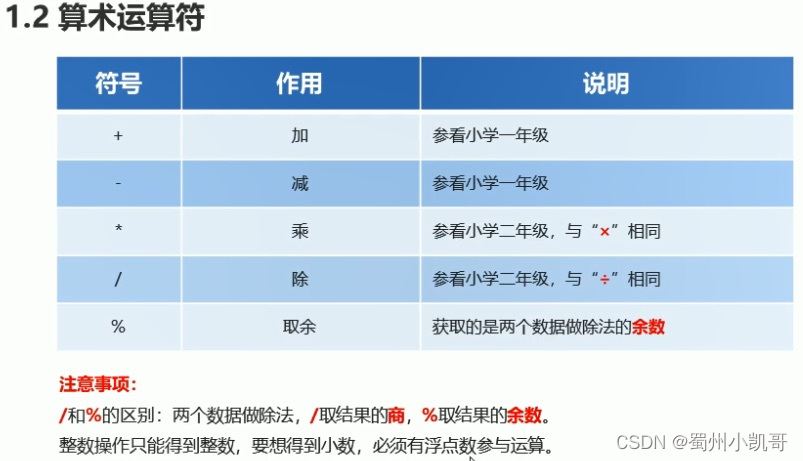2、运算符号与表达式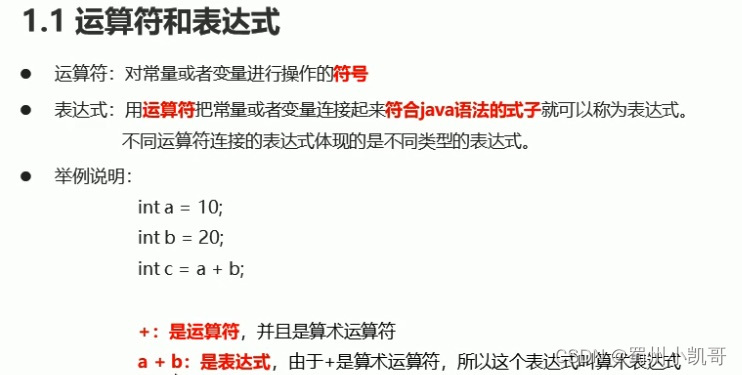3、字符的“+”操作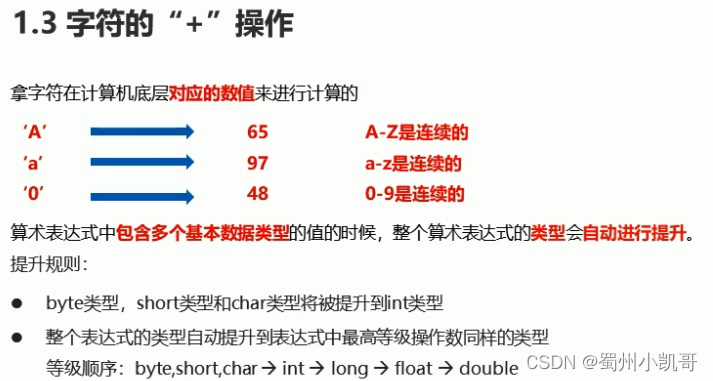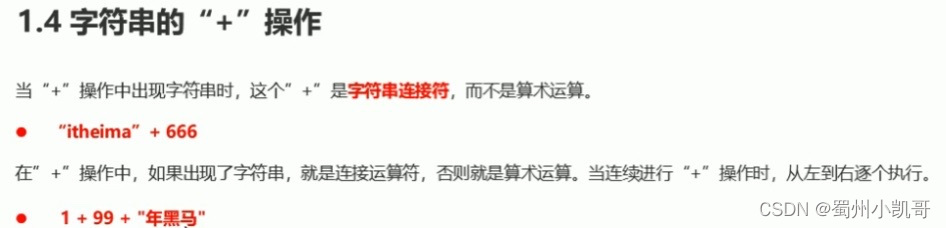注： += 隐含了类型强转

例如：short s = 10;

s += 20; 结果就是30

s = s+20; 编译时就会报错类型不兼容

4、赋值运算符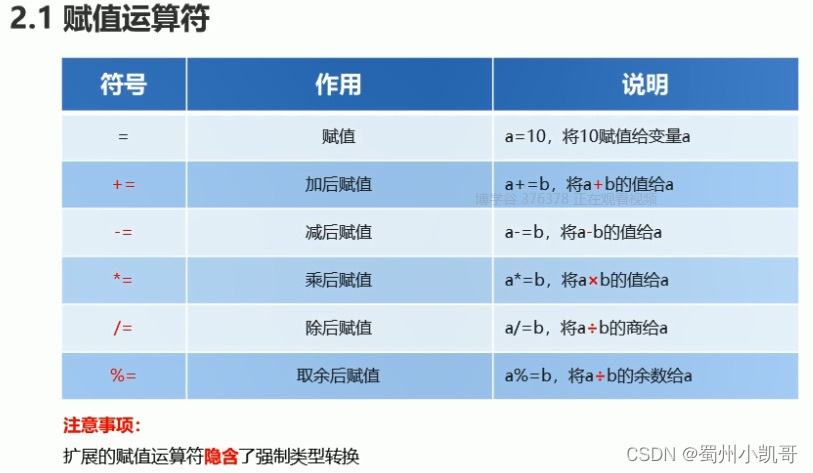5、自增自减运算符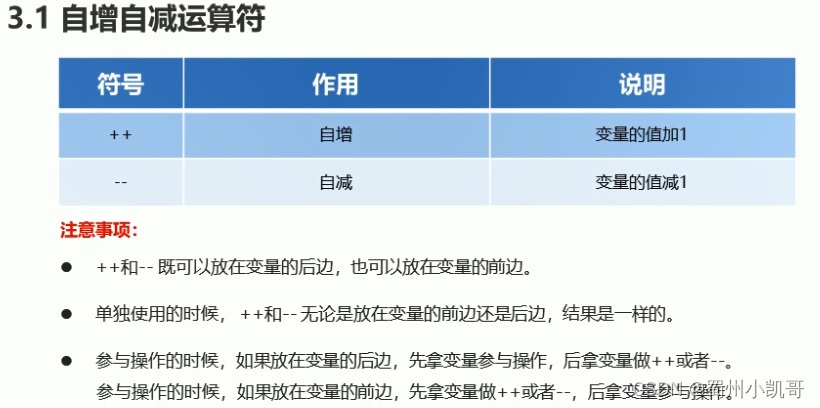注：自增、自减运算符合++、-- 参与运算时

++、-- 在前，先自加自减再赋值

++、-- 在后，先赋值再自加自减

6、关系运算符：返回的结果都是布尔值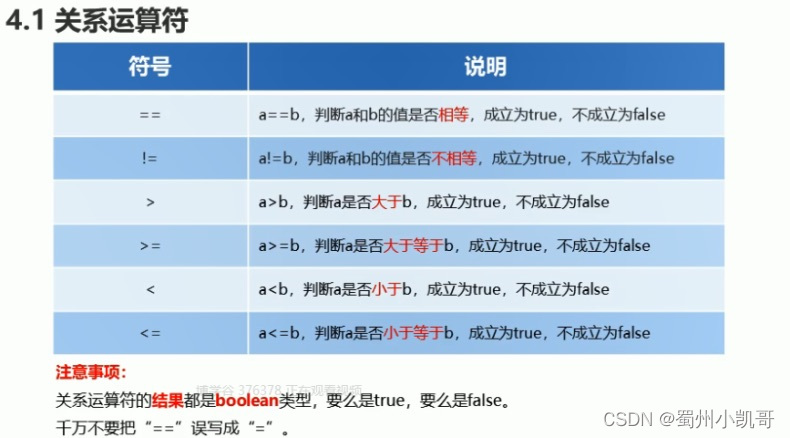逻辑运算符

1、逻辑运算符分类及介绍

逻辑与 & : 表达式两边都是true结果为true,表达式中只要有一边为false结果都为false（有false则false）

逻辑或 | ：表达式中只要有一边为true结果为true,表达式中两边结果都为false结果都为false（有true则true）

逻辑非 ! ：取反原则，! ture结果为false,! false结果为true

逻辑异或 ^ ：表达式两边都是true/false结果都为false,表达式中只要有一边为true结果都为true(相同为false，不同为true)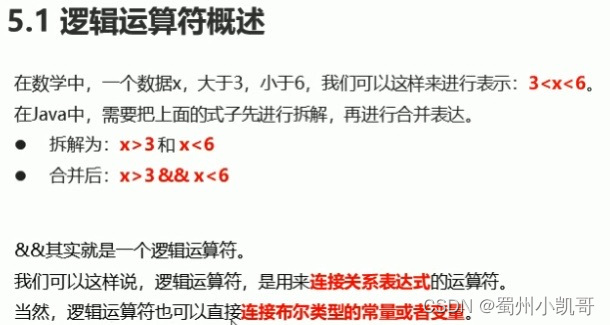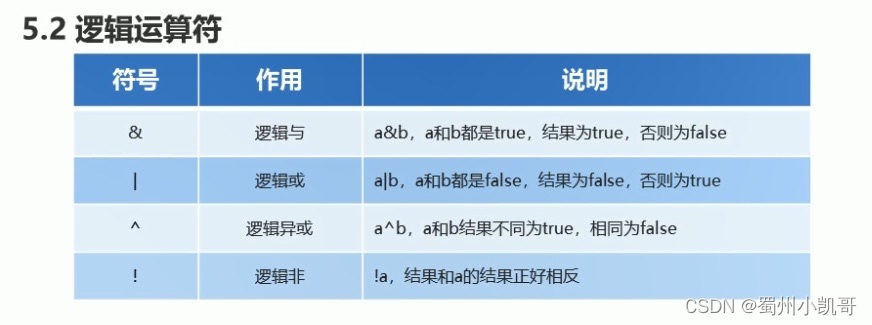2、短路运算符两个：&&、||

短路与&& : （结果：有false则false），区别&：&两边的表达式都要运算，&&只要左边为false时右边不运算

短路或|| : （结果：有true则true），区别 | : |两边的表达式都要运算，||只要左边为true时右边不运算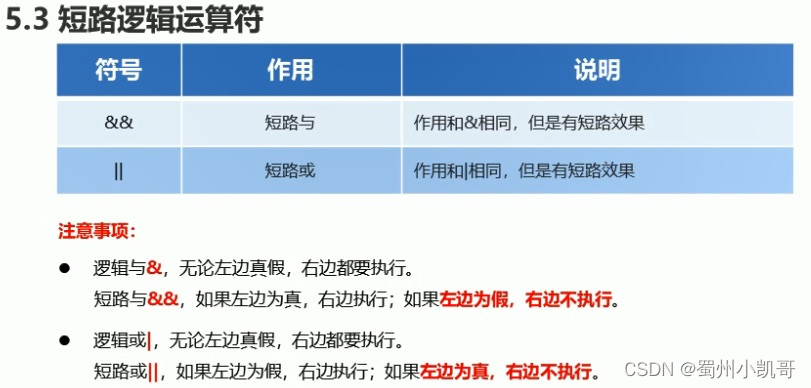3、三元运算符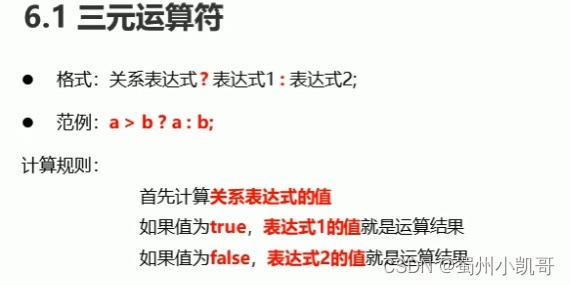展开全文java
• 【C语言/java基础】C语言基础算术运算符，赋值运算符关系运算符，逻辑运算符，位运算符，三目运算符，最详细的计算方法和求解步骤。
• JavaScript运算符算术运算符赋值运算符关系(比较)运算符逻辑运算符运算符的优先级 算术运算符 算术运算符用在数学表达式中, 它的使用方式和数学中也是一致的. 算术运算符是对数据进行计算的符号 可以直接对数据进行...运算符
• 算术运算符 除 /： 1、参与的数类型都是整型时，做取整运算 即商n余m，结果为n 2、只要有一个浮点数参与，就会做类似精确运算 ##取余%： 取余运算符号，也叫取模运算符号 做除法运算时，商n余m，结果为m，...运算符优先级 运算符 执行顺序
• 目录1 运算符 种类1.1算数运算符1.2赋值运算符1.3关系运算符1.4逻辑运算符1.5三目运算符2 运算符 优先级 1 运算符 种类 1.1算数运算符 Column 1 Column 2 centered 文本居中 right-aligned 文本居右 1.2...java
• 关系运算符 “>、<、>=、<=、==、!=” 用于判断两个数据之间的大小关系，计算结果为boolean,如果成立返回为true，否则为false. double d1=10; System.out.println(d1>3); //返回true ==和=的区别: ...java 编程语言
• 赋值、关系运算符 一、赋值运算符 (一)、基本的赋值运算符是=,一开始可能会以为它是“等于”，其实不是的,可以读作"等于" (二)、赋值运算符实际上意味着把等号右边的值或表达式的值赋给等号左边 (三)、运算符 1、+=...
• 按位“”/赋值 * 乘法 *= 乘法/赋值 + 添加 += 加法/赋值 – 减法 –= 减法/赋值 < 小于 << 左移 <<= 左移/赋值 <= 小于或等于 = 赋值 == 相等 > 大于 >= 大于或...
• Python同其他语言一样，赋值运算符主要用来为变量进行赋值。Python中，变量不用先定义，可以直接使用，即在使用时，可以直接把基本赋值运算符“=”右边的值赋给左边的变量，也可以进行某些运算后再赋值给左边的变量...
• 赋值运算符、算术运算符、关系运算符、逻辑运算符、条件运算符（三元运算符）、位运算符、运算符的优先级java
• 一、赋值运算符与赋值表达式 1、赋值表达式是由赋值运算符“=”将一个变量和一个表达式连接起来的式子，其一般格式： 变量=表达式 表示将“=”右边表达式的值赋给左边的变量，表达式可以是符合C语言语法的各种...
• short s = 1; s = s + 1; ** short s = 1; s += 1; 以上两种写法结果分别是？第一种写法会报错，原因是short类型int类型做运算，会向上转型为int类型，最后将int类型的值直接赋值给short类型会报错。
• 运算符分为二元运算符关系运算符赋值运算和三者表达式。下面分别记录运算符的运算规则和示例 二元运算符分为乘法(*)、加法(+)、减法(-)、除法(/)、取余(%) 前面四种和我们数学上面的基本一致,运算从左往右计算，...
• 最容易混淆的是= 对于 它属于关系运算符，用于比较运算，包括大于，小于 对于= 它属于赋值运算符，用于赋值运算。...而一般例如if()，while()，for()中括号里的是关系运算符，不是赋值运算符。 ...
• 逻辑运算符： && ： 条件1 条件2，两个条件同时满足 就是为true || ：或 条件1 或 条件2 ，有一个满足就为true ...赋值运算符： += ： -= ： *= ： /= ： %= ： #include int main() { int i=3; //i
• Python学习（3）算数运算符、赋值运算符、比较（关系）运算符 运算符是一些特殊的符号，主要用于数学运算，比较大小和逻辑运算等。 3.1算数运算符 算数运算符号是处理四则运算的符号，常用的算数运算符如表： 算数...python 编程语言
• 赋值运算符 赋值运算符 ...关系运算符 关系（比较）（结果值一定是布尔值） == ！= >= <= > < 算术运算符 算数运算符 + - * / % ++ -- ++ --： 使用方式：单独使用 参与运...java
• 在程序中经常需要比较两个数据的大小，以决定程序下一步的工作。比如一个程序限制了只能成年人使用，儿童因为年龄不够，没有...关系运算符的优先级低于算术运算符，高于赋值运算符。在六个关系运算符中，<、<=、>
• Python算术运算符 以下假设变量a为10，变量b为21： 运算符 描述 实例 + 加：两个对象相加 a + b = 31 - 减：得到负数或是一个数减去另一个数 a - b = -11 * 乘：两个数相乘或是返回一个被重复若干次的...python
• 一，运算符(7种算数运算符，比较运算符，赋值运算符，成员运算符，身份运算符，逻辑运算符，位运算符) 优先级： (1) 个别运算符: 优先级最高 ** 幂运算 优先级最低 = 赋值运算符 () 括号可以提升运算优先级 (2)...python
• ## C语言赋值运算符和赋值表达式

千次阅读 多人点赞 2021-08-04 16:09:48
C语言赋值运算符和赋值表达式赋值运算符类型转换复合的赋值运算符 赋值运算符 简单赋值运算符和表达式:简单赋值运算符记为“=”。由“= ”连接的式子称为赋值表达式。其一般形式为： 变量=表达式 例如： x=a+b w=...
• C语言赋值运算符与赋值表达式我们都知道基本的赋值运算符是"="，他的优先级别低于其他的运算符，所以对该运算符往往最后读取。下面和大家分享一下C语言赋值运算符与赋值表达式，一起来看看吧！一、赋值运算符与赋值...
• 运算符优先级：同一表达式中多个运算符被执行的次序，在表达式求值 时，先按运算符的优先级由高到低的次序执行。 括号()具有最高优先级，编写时可多加利用。java
• 算术运算符及赋值运算符 1 )算术运算符  例: 5 + 3 10-6 3*4 8/2 10%3 这样的用算术运算符结合起来的式子，我们就称之为算术表达式。 2 )...
• 关系运算符 逻辑运算符 位运算符 赋值运算符 其他运算符 2. 算术运算符 下表列出了所有Go语言的算术运算符。假定 A 值为 10，B 值为 20。 运算符 描述 实例 + 相加 A + B 输出结果 30 - 相减 A - B 输出...Go 运算符
• 一、算数运算符 二、关系运算符 三、逻辑运算符 四、位运算符 五、赋值运算符 六、其他运算符 七、运算符优先级python 编程语言 pycharm...